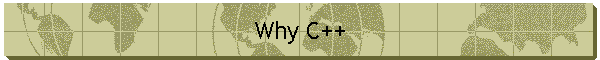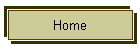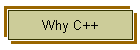# Why I Chose C++

#### by William T. Block

A good way to get into an argument with a computer programmer it to attempt to explain why the language they are using is not as good as the one you are using.  Most of the programmers I know are positively religious over their Operating System (see Why I Chose Windows), their development language and finally their text editor.

As you might have inferred by the title, I feel that C++ is the superior computer programming language.  I will begin by qualifying that statement somewhat.  I learned to program using Pascal and I still feel that it is a good language for learning computer programming.   Pascal is type safe, has a very limited number of keywords and encourages good programming principals. The BASIC language in the form of Visual Basic on the other hand is an ideal language for quickly putting together a project and for taking advantage of data base access, and other advanced programming tools such as Microsoft's Component Object Model (COM).

Why is it then that modern operating systems and very large applications are written in C++? VisualBasic and Pascal (in the form of Delphi) barely resemble the ANSI definition of these languages.  Each of these languages are proprietary and lock you in to a particular vendor.  This does not particularly bother me, since I program exclusively for the Windows environment and use Microsoft's Visual C++ as my development environment.   There are other features of C++ that are attractive when doing medium to large scale development: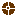Operator overloading (also in Delphi)Exceptions (also in Delphi and Java)Templates

These are primary features of C++ that set it apart from its peers.  I am not going to mention Object Oriented Programming (OOP) here because Java, VB and Delphi support OOP in some form or fashion.  I would place OOP above the other three bullets if I were including it in this discussion.

## Operator Overloading

I am sure that many of my C++ peers would disagree with me about the relative importance of the three features that I have selected.  Operator overloading is essential to making a library of code "fool proof."  First lets look at a typical example of operator overloading:

class CVeryLong
{
public:
// default contructor inits member variables to zero

CVeryLong(){ m_lHigh = m_lLow = 0; }
// initialization constructor sets member variables to a value
CVeryLong( long lHigh, long lLow ){ m_lHigh = lHigh; m_lLow = lLow; }
virtual ~CVeryLong(){}; // destructor
void SetHighValue( long lValue ){ m_lHigh = lValue; }
long GetHighValue(){ return m_lHigh; }
void SetLowValue( long lValue ){ m_lLow = lValue; }
long GetLowValue(){ return m_lLow; }
BOOL operator < ( CVeryLong& refValue )    // less than operator
{
if ( m_lHigh < refValue.GetHighValue()) return TRUE;
else if ( m_lHigh > refValue.GetHighValue()) return FALSE;
else if ( m_lLow < refValue.GetLowValue()) return TRUE;
else return FALSE; // >=
}
BOOL operator > ( CVeryLong& refValue )    // greater than operator
{
if ( m_lHigh > refValue.GetHighValue()) return TRUE;
else if ( m_lHigh < refValue.GetHighValue()) return FALSE;
else if ( m_lLow > refValue.GetLowValue()) return TRUE;
else return FALSE; // <=
}
BOOL operator == ( CVeryLong& refValue ) // equivalence operator
{
return m_lHigh == refValue.GetHighValue() && m_lLow == refValue.GetLowValue();
}

private:
long m_lLow;
long m_lHigh;
};

The CVeryLong class keeps two private long variables to represent a single 64 bit integer.  In this class we are overloading the less than (<), greater than (>) and equivalence (==) operators to allow comparison of our new 64 bit integer class.  This class is obviously not complete since I have not overloaded other key operators such as the arithmetic operators.  Here is some sample code using our new class:

{   CString csText;

CVeryLong vl1( 1, 2 ), vl2( 1, 3 ), vl3;

cout << "vl1 is (1, 2)" << endl;
cout << "vl2 is (1, 3)" << endl;
cout << "vl3 is (1, 2)" << endl;

csText = "vl1 < vl2 is ";
csText += vl1 < vl2 ? "true" : "false";
cout << (LPCTSTR)csText << endl;

csText = "vl1 > vl2 is ";
csText += vl1 > vl2 ? "true" : "false";
cout << (LPCTSTR)csText << endl;

csText = "vl1 == vl2 is ";
csText += vl1 == vl2 ? "true" : "false";
cout << (LPCTSTR)csText << endl;

csText = "vl1 < vl3 is ";
csText += vl1 < vl3 ? "true" : "false";
cout << (LPCTSTR)csText << endl;

csText = "vl1 > vl3 is ";
csText += vl1 > vl3 ? "true" : "false";
cout << (LPCTSTR)csText << endl;

csText = "vl1 == vl3 is ";
csText += vl1 == vl3 ? "true" : "false";
cout << (LPCTSTR)csText << endl;
}

Running the program containing the above code generates the following output:

vl1 is (1, 2)
vl2 is (1, 3)
vl3 is (1, 2)
vl1 < vl2 is true
vl1 > vl2 is false
vl1 == vl2 is false
vl1 < vl3 is false
vl1 > vl3 is false
vl1 == vl3 is true

At this point we have told the compiler what to do when it sees the >, < or == operators used with our class.  We could have just as easily defined a GreaterThan, LessThan and EqualTo member function to do the same thing -- and in other languages this is exactly what you would have to do:

vl1 == vl3 would be equivalent to vl1.EqualTo( vl3 )

So we have made the notation more concise and consistent with the intrinsic data types, but when we began this discussion I stated that operator overloading would make the code "fool proof."  Notice that in the middle of the sample usage code:

vl3 = vl1; // assign vl1 to vl3 and do the comparisons again

We did not overload the assignment operator, so C++ behaves like most languages when presented with this statement and simply copies the member variables from one object to the other.  What if our class were going to support n-level precision so that at compile time we do not know how many longs to allocate?  We would have to dynamically allocate the variables in the constructor and free them in the destructor.  Our member variables might look like this:

private:
short m_nValues; // number of values allocated
long* m_pValues; // array of values

The default assignment operator will copy the member variables just as it did before, only now you have two pointers pointing to the same block of allocated memory.  When the destructor for the first object runs, it will free the memory block with no problems, but when the second object's destructor runs, it tries to free the same block of memory -- bug and crash.  Even though you can write a Copy function to do the right thing, you cannot keep some unsuspecting programmer from coding the assignment that "works most of the time."  VisualBasic is still "fool proof" at this point because it does not allow dynamic memory allocation, but Pascal (not Delphi) will let you crash and burn at this point.

In C++ the assignment operator would be written as follows:

CVeryVeryLong operator = ( CVeryVeryLong& refValue ) // assignment operator
{

delete [] m_pValues; // free previous values
m_nValues = refValue.GetNumberOfValues(); // needs to be defined
m_pValues = new long[ m_nValues ]; // allocate new values
// GetBuffer() in the following line needs to be defined
memcpy( m_pValue, refValue.GetBuffer(), sizeof(long) * m_nValues ); // copy the array contents
return *this;
}

The conclusion here is that a language should either cripple itself as is the case with Java or VB (no dynamic memory allocation) or provide the programmer with a way of guaranteeing that users of your code cannot use it improperly.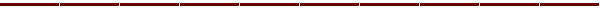## Exceptions

Exceptions provide another capability that is hard to duplicate if not supported by the language.  Windows NT provides a sophisticated capability called Structured Exception Handling (SEH) where the OS is providing the same type of functionality that is provided by C++. It may be possible to take advantage of SEH in other languages.   C++ provides a portable mechanism that will work in all operating systems.

Exception handling is exactly what the name implies -- the ability to handle exceptional cases without having to tax the normal case.  Look at this example of exception processing:

DWORD dwStart = ::GetTickCount(); // used for timing in mSec
const int x = 1000000;
const int xEnd = -x;
int y = x;
int z;
while ( y > xEnd )
{
try
{
while ( y > xEnd )
z = x / y--; // divide protected by exception
}
catch (...)
{
cout << "Divide by zero" << endl; // trapped out of inner loop
y--; // continue via outer loop
}
}
DWORD dwStop = ::GetTickCount();
DWORD dwDiff = dwStop - dwStart;
CString csMessage;
csMessage.Format( "mSec = %d", dwDiff );
cout << (LPCTSTR)csMessage << endl;

The output from this code looks like this:

Divide by zero
mSec = 491

The inner loop is free to run without having to do a test for zero on every iteration -- the divide by zero is handled as an exceptional case instead of having to treat is as the "rule."  Here is how this condition could be handled without exceptions:

while ( y > xEnd )
{
if ( x != 0 ) // has to execute for every
iteration
{    z = x / y--;
} else
{     cout << "Attempted divide by zero" << endl;
}
}
The output from this code looks like this:

Attempted divide by zero
mSec = 570

My work involves signal processing in real-time, where every millisecond counts. In the above example, the software could process 2 million integer divides without having to explicitly check for 2 million divide errors, by treating the divide by zero as an "exceptional case."## Templates

While templates may not be able to make your code "fool proof" as can overloaded operators or faster and more robust by handling exceptions, they are my favorite C++ feature.  Templates can save the programmer from having to write a lot of code and they benefit the final product by making it smaller.

Templates are type safe macros that are built on demand, that is whatever part of the template is not used is not included in the code. There are class templates and function templates.

### Function Templates

With function templates, you can specify a set of functions that are based on the same code, but act on different types or classes. Here is an example of a function template that returns the maximum of two values:

template <class T> T& Max( T& a, T& b )
{
if ( a > b ) return a; else return b;
}

This is a data type independent way of comparing two floats, two ints, two shorts, two chars, etc. without having to write the MaxFloat, MaxInt, MaxShort, MaxChar, etc.  Furthermore, if I only use the float in my current application, no code is generated for anything else. If I invoke Max with a float and a char, the compiler will complain.  Here is an example use:

int n1 = 5, n2 = 10, n3;
float f1 = 5.1f, f2 = 10.5f, f3;
CVeryLong vl1( 1, 2 ), vl2( 2, 15 ), vl3; // remember CVeryLong?

n3 = Max( n1, n2 );
f3 = Max( f1, f2 );
vl3 = Max( vl1, vl2 );

CString csMessage;
csMessage.Format( "n3 = %d, f3 = %f, vl3 = (%d, %d)",
n3, f3, vl3.GetHighValue(), vl3.GetLowValue() );
cout << (LPCTSTR)csMessage << endl;

The output generated is as follows:

n3 = 10, f3 = 10.500000, vl3 = (2, 15)

Notice that we are not limited to intrinsic types because of operator overloading.   Since our CVeryLong class overloads the greater than (>) operator, it can be used with the Max template as well.  Template libraries like the Standard Template Library (STL) and the Active Template Library (ATL) are famous for generating tiny code and being highly re-useable.

If you use another language, imagine what is involved to provide something as simple as the Max template while making it applicable to any class that you define, and also to any class that the user's of your library define!

### Class Templates

You can use class templates to create a family of classes that operate on a type. Look at this example of a balanced binary tree class:

template<class KEY, class ARG_KEY, class DATA, class ARG_DATA>
class CTree
{
private:
typedef enum
{   balLeft = -1,
balEven,
balRight,
} BALANCE;

class CNode;
typedef CNode* PNODE;

class CNode // container to hold the data and hide the balancing details
{
public:
CNode( ARG_KEY key, ARG_DATA data );

// additional members not shown
private:
KEY m_key;
DATA m_data;
BALANCE m_Bal; // -1..1 current balance data for this node
PNODE m_pLeft;
PNODE m_pRight;
};

CTree(){ m_pRoot = 0; m_nSize = 0; m_bHeightChange = false; }
virtual ~CTree();
bool GetFirst( ARG_KEY key, ARG_DATA data );
bool GetLast( ARG_KEY key, ARG_DATA data );
bool GetNext( ARG_KEY key, ARG_DATA data );
bool GetPrev( ARG_KEY key, ARG_DATA data );
bool Add( ARG_KEY key, ARG_DATA data );
ARG_DATA operator[]( ARG_KEY key );
bool Delete( ARG_KEY key );

// additional members not shown

private:
CNode* m_pRoot;
bool m_bHeightChange;
int m_nSize;
};

I have specifically used a complex example here, to drive home the point that templates can save you a lot of programming.  If you have ever coded a balanced binary tree, you know that it is difficult, and depending on the programming language, messy code to write.  I have personally had the pleasure of coding such a library three times in three different languages: C, Pascal and C++.  In C and Pascal I was able to use untyped pointers to allow the tree to hold any data type, but in both of these cases I limited the key to be a string.

The C++ implementation in the above example can contain any data type,  can use any data type for the key, and the implementation is completely type safe!

Lets examine the template's declaration:

template<class KEY, class ARG_KEY, class DATA, class ARG_DATA>
class CTree

The four arguments in the declaration give the data type of the key, how the key is passed into arguments, the data type of the data the tree will contain, and how the data is passed into arguments. Here are some examples of how the CTree could be instantiated:

CTree<CString, const char*, CVeryLong, CVeryLong&> treeVeryLong;
CTree<CString, const char*, float, float> treeFloat;
CTree<long, long, CString, const char*> treeString;
CTree<CVeryLong, CVeryLong, CList<int, int>, CList<int, int>&> treeList;

The first case is a tree of CVeryLong's using a CString as the key.  The key is passed into arguments as a const char* and the data is passed into arguments using a reference to CVeryLong.

The second case is similar to the first except the data is a float type.

The third case is a tree of strings that use a long for the key.

In the forth case, a tree of linked lists of integers (another class template) is using CVeryLong types for the key.

We could use the treeVeryLong template to keep track of programmer salaries:

treeVeryLong.Add( "Ray", CVeryLong( 100, 50 ));
treeVeryLong.Add( "Barb", CVeryLong( 200, 25));

// Note that the bracket operator [] was overloaded in our CTree class
// to allow following type of addressing of our tree, and the greater than
// operator of the CVeryLong class enables the comparison

if ( treeVeryLong[ "Barb" ] > treeVeryLong[ "Ray" ] )
{    cout << "Barb is the best!" << endl;
} else
{    cout << "Ray is the best!" << endl;
}

Actually there is an error in this code -- do you see it?  We specified in our template declaration that the ARG_DATA parameter was to be a reference to a CVeryLong (ARG_DATA CveryLong&).  Since we are passing in a constant here, the compiler will complain. We can correct this error by changing the template declaration to allow data arguments to be passed in by value, or modify the code as follows:

CVeryLong vlRay( 100, 50 );
CVeryLong vlBarb( 200, 25 );
treeVeryLong.Add( "Ray", vlRay );
treeVeryLong.Add( "Barb", vlBarb );

Class templates provide a powerful code reuse mechanism that can save a tremendous amount of programming. In addition to saving development time, use of templates will generally result in much smaller projects.  Microsoft ships two libraries with Visual C++: Microsoft Foundation Class (MFC) and the Active Template Library (ATL).  ATL was developed in response to the advent of the internet, more specifically to the large number of people with slow access (read 28.8k) to the internet.  MFC projects were too large to be practical for creating web page objects that had to be downloaded to the client's computer.  ATL was the solution to this problem -- projects based on ATL are very small and quick to download.## Conclusion

I readily admit to being a C++ bigot, but it is bigotry born of experience with several languages, operating systems, and Windowing systems.  Programming code in C++ is a pleasure and I would not look forward to having to return to the old days without operator overloading, exceptions, and templates.